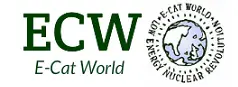# Rossi ‘Thinks’ Zero Point Energy is Source of E-Cat’s Power

Here’s an interesting Q&A from the Journal of Nuclear Physics today:

Greta
July 16, 2020 at 1:47 AM
Dr Rossi,
Does have your effect in the Ecat SKL its primary source in the point zero energy ?
Greta

Andrea Rossi
July 16, 2020 at 2:46 AM
Greta:
I think so,
Warm Regards,
A.R.

A summary about zero-point energy (ZPE) from Wikipedia:

Zero-point energy (ZPE) is the lowest possible energy that a quantum mechanical system may have. Unlike in classical mechanics, quantum systems constantly fluctuate in their lowest energy state as described by the Heisenberg uncertainty principle. As well as atoms and molecules, the empty space of the vacuum has these properties. According to quantum field theory, the universe can be thought of not as isolated particles but continuous fluctuating fields: matter fields, whose quanta are fermions (i.e., leptons and quarks), and force fields, whose quanta are bosons (e.g., photons and gluons). All these fields have zero-point energy. These fluctuating zero-point fields lead to a kind of reintroduction of an aether in physics, since some systems can detect the existence of this energy; however, this aether cannot be thought of as a physical medium if it is to be Lorentz invariant such that there is no contradiction with Einstein’s theory of special relativity.

Physics currently lacks a full theoretical model for understanding zero-point energy; in particular, the discrepancy between theorized and observed vacuum energy is a source of major contention. Physicists Richard Feynman and John Wheeler calculated the zero-point radiation of the vacuum to be an order of magnitude greater than nuclear energy, with a single light bulb containing enough energy to boil all the world’s oceans

https://en.wikipedia.org/wiki/Zero-point_energy

ZPE is one of those fields that has been discussed and theorized in the world of science (and science fication) and has fascinating possibilities, but to this point, no technology has come to market that harnesses ZPE, but maybe if Rossi is correct, the E-Cat can change all that.